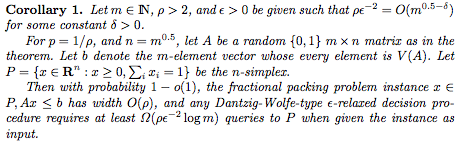## neal young / Klein15Number

•This paper gives a lower bound on the complexity of $$(1 \pm \epsilon)$$-approximately solving a packing or covering problem using a certain class of Lagrangian-relaxation algorithms: any such algorithm, given a problem formed by a random {0,1}-matrix, requires (with high probability) a number of iterations proportional to $$(\rho \epsilon)^2\log m$$. (Here $$\rho$$ is a technical parameter, thewidth'' of the problem instance.)

The class of algorithms in question includes Dantzig-Wolfe decomposition, Benders decomposition, the Lagrangian-relaxation method developed by Held and Karp  for lower-bounding TSP, and algorithms recently studied by many authors (including Serge Plotkin, David Shmoys, and Eva Tardos ; Mike Grigoriadis and L.G. Khachiyan ; Michael Luby and Noam Nisan ; Naveen Garg and Jochen Konemann ; and Lisa Fleischer ). The lower bound matches the known upper bounds within a constant factor. The lower bound is useful because, in practice, the dependence on $$\epsilon$$ limits the applicability of these algorithms. The lower bound provides some insight into what is necessary to surmount this dependence.
Journal version of . Published online Aug. 2015.